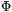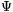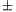Mathematical and Physical Journal
for High Schools
Issued by the MATFUND Foundation
 Already signed up? New to KöMaL?

#Problem A. 517. (October 2010)

A. 517. Let m3 be a positive integer, and letm(x) be the mth cyclotomic polynomial, and denote bym(x) the polynomial with integer coefficients for which. Prove that for every integer a, any prime divisor of the numberm(a) either divides m or is of the form mk1.

(5 pont)

Deadline expired on November 10, 2010.

### Statistics:

 5 students sent a solution. 5 points: Backhausz Tibor. 4 points: Ágoston Tamás, Nagy 235 János, Nagy 648 Donát. 3 points: 1 student.

Problems in Mathematics of KöMaL, October 2010